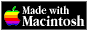THE HYPERCARD CENTER

 HyperTalk Reference
 Home » HyperTalk Reference » Operators and constants Select a destination << Home Stack < HyperTalk Reference Arithmetic operatorsComparison operatorsLogical operatorsString operatorsType and existence operatorsConstants

# Comparison operators

### =, is

Results in` true `if the expression to its left and the expression to its right have the same values. The expressions can be arithmetic, text, or logical.

```N = 0 word 1 of card field 1 = 10 the name of this card is "Index" ```

### <>, ≠, is not

Results in` true `if the expression to its left and the expression to its right have different values. The expressions can be arithmetic, text, or logical.

```the number of this card <> 1 the number of this card ≠ 1 the name of this card is not "Index" ```

Note: To get the character` ≠`, hold down Option and press` =`.

### <

Results in` true `if the expression to its left has a value less than the one to its right. The expressions can be both arithmetic or both text.

```the number of this card < 10 the freesize of this stack < 5120 ```

### >

Results in` true `if the expression to its left has a value greater than the one to its right. The expressions can be both arithmetic or both text.

```23 > 13 the version > 1.2.5 (the freesize of this stack) > 10240 ```

### <=, ≤

Results in` true `if the expression to its left has a value less than or equal to the one to its right. The expressions can be both arithmetic or both text.

```the number of this card ≤ 10 the freesize of this stack <= 5120 ```

Note: To get the character` ≤`, hold down Option and press` <`.

### >=, ≥

Results in true if the expression to its left has a value greater than or equal to the one to its right. The expressions can be both arithmetic or both text.

```23 >= 13 the version ≥ 1.2.5 (the freesize of this stack) ≥ 10240 ```

Note: To get the character `≥`, hold down Option and press `>`.

### Contains

Results in true if the text string yielded by the expression on its right is found in the text string yielded by the expression on its left (the converse of the operator` is in`).

```"hello world" contains "lo wo" the name of this card contains "operator" ```

### is in

Results in` true `if the text string yielded by the expression on its left is found in the text string yielded by the expression on its right (the converse of the operator` contains`).

```"lo wo" is in "hello world" "operator" is in the name of this card ```

### is not in

Results in` true `if the text string yielded by the expression on its left is not found in the text string yielded by the expression on its right (the opposite of the operator` is in`).

```"Apple" is not in "oranges" "operator" is not in the name of this card ```

### is within

Results in` true `if the point yielded by the expression on its left is contained within the rectangle yielded by the expression on its right.

```"20,20" is within "0,0,40,40" the mouseLoc is within the rect of ¬ card button 1 ```

### is not within

Results in` true `if the point yielded by the expression on its left is not contained within the rectangle yielded by the expression on its right.

```"20,20" is not within "0,0,15,15" the mouseLoc is not within the rect of ¬ card button 1 ```

## Related Topics

Version 0.7b1 (March 24, 2022)Switch to Modern View